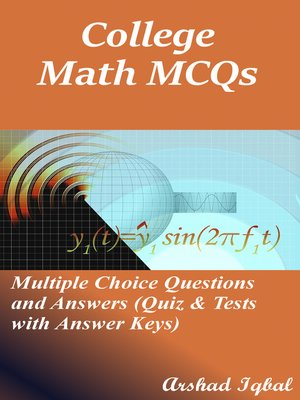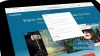# College Math MCQs

### Multiple Choice Questions and Answers (Quiz & Tests with Answer Keys)

ebook

With an OverDrive account, you can save your favorite libraries for at-a-glance information about availability. Find out more about OverDrive accounts.

### Subjects

"College Math MCQs: Multiple Choice Questions and Answers (Quiz & Tests with Answer Keys)" provides practice tests for competitive exams. "College Math MCQ" will help with theoretical, conceptual, and analytical study for self-assessment, career tests. This book can help to learn and practice college math quizzes as a quick study guide for placement test preparation.
"College Math Multiple Choice Questions and Answers (MCQs)" pdf is a revision guide with a collection of trivia questions to fun quiz questions and answers pdf on topics: Application of basic identities, double angle identities, functions and limits, fundamentals of trigonometry, matrices and determinants, number system, partial fractions, permutations, combinations and probability, quadratic equations, sequences and series, sets, functions and groups, trigonometric functions and graphs, trigonometric identities, trigonometric ratios of allied angles to enhance teaching and learning.
College Math Quiz Questions and Answers pdf also covers the syllabus of many competitive papers for admission exams of different universities from math textbooks on chapters:

Application of Basic Identities Multiple Choice Questions: 20 MCQs. Double Angle Identities Multiple Choice Questions: 19 MCQs. Functions and Limits Multiple Choice Questions: 125 MCQs. Fundamentals of Trigonometry Multiple Choice Questions: 78 MCQs. Matrices and Determinants Multiple Choice Questions: 26 MCQs. Number System Multiple Choice Questions: 68 MCQs. Partial Fractions Multiple Choice Questions: 36 MCQs. Permutations, Combinations and Probability Multiple Choice Questions: 69 MCQs. Quadratic Equations Multiple Choice Questions: 76 MCQs. Sequences and Series Multiple Choice Questions: 79 MCQs. Sets, Functions and Groups Multiple Choice Questions: 30 MCQs. Trigonometric Functions and Graphs Multiple Choice Questions: 42 MCQs. Trigonometric Identities Multiple Choice Questions: 125 MCQs. Trigonometric Ratios of Allied Angles Multiple Choice Questions: 10 MCQs.

The chapter "Application of Basic Identities MCQs" covers topics of applied mathematics, and trigonometry basics.
The chapter "Double Angle Identities MCQs" covers topic of double angle identities.
The chapter "Functions and Limits MCQs" covers topics of introduction to functions and limits, exponential function, linear functions, logarithmic functions, concept of limit of function, algebra problems, composition of functions, even functions, finding inverse function, hyperbolic functions, inverse of a function, mathematical formulas, notation and value of function, odd functions, parametric functions, and trigonometric function.
The chapter "Fundamentals of Trigonometry MCQs" covers topics of trigonometric function, fundamental identities, trigonometry formulas, algebra and trigonometry, mathematical formulas, measurements conversion, measuring angles units, radian to degree conversion, radians to degrees, and trigonometry problems.
The chapter "Matrices and Determinants MCQs" covers topics of introduction to matrices and determinants, rectangular matrix, row matrix, skew-symmetric matrix, and symmetric matrix, addition of matrix, adjoint and inverse of square matrix, column matrix, homogeneous linear equations, and multiplication of a matrix.
The chapter "Number System MCQs" covers topics of properties of real numbers, rational numbers, irrational numbers, complex numbers, basic function, binary operation, De Moivre's theorem, groups, linear and quadratic function, sets, operation on three sets, and relation.
The chapter "Partial Fractions MCQs" covers topics of introduction of partial fractions, rational fractions, resolution of a rational...

### Publication Details

Publisher:
Imprint:
Smashwords Edition
Publication Date:
2016

### Format